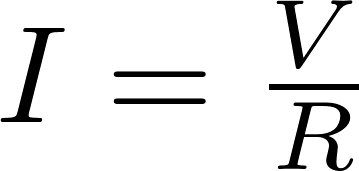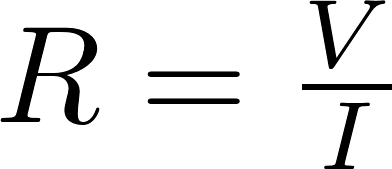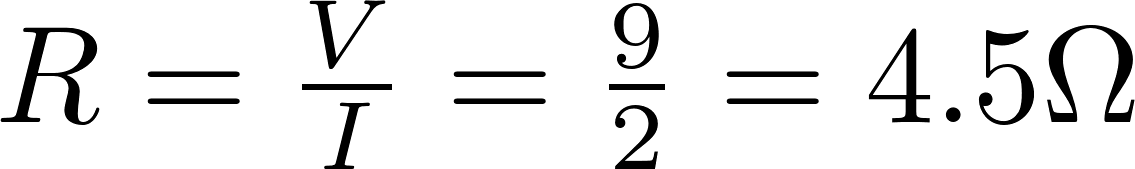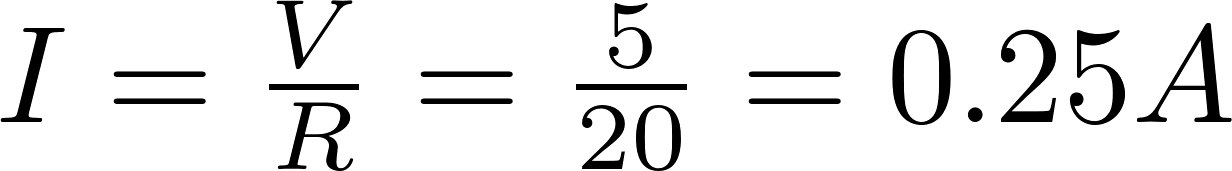# Ohm's law

Ohm's law describes the relation between Voltage, Current and Resistance. The law states that the voltage is equal to the current multiplied by the resistance. Ohm's law is named after the German physicist Georg Ohm.

### Formulas

#### Voltage

The voltage can be calculated by multiplying the current by the resistance:#### Current

The current can be calculated by dividing the voltage by the resistance:#### Resistance

The resistance can be calculated by dividing the voltage by the current:#### Power

The power can be calculated by multiplying the voltage by the current:V is the symbol for voltage and is measured in volt (V).
I is the symbol for current and is measured in ampere (A).
R is the symbol for resistance and is measured in ohm (Ω).
P is the symbol for power and is measured in watt (W).

### Calculator

Enter two values to calculate the remaining two.

#### Example 1

Bob has a circuit connected to a 9 volt battery and measures a current of 2 amps. What is the resistance in this circuit?#### Example 2

Alice's circuit is connected to a 5 volt battery and measures a resistance of 20 ohms. Calculate the power in this circuit.

We can first calculate the current:We can now calculate the power